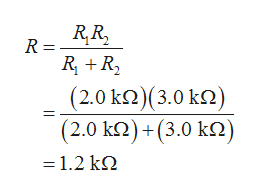Question
2 views

The circuit in Figure P18.62 contains two resistors, R1 = 2.0
kΩ and R2 = 3.0 kΩ, and two capacitors, C1 = 2.0 µF and C2 =
3.0 µF, connected to a battery with emf ε = 120 V. If there are
no charges on the capacitors before switch S is closed, determine
the charges q1 and q2 on capacitors C1 and C2, respectively,
as functions of time, after the switch is closed. Hint: First
reconstruct the circuit so that it becomes a simple RC circuit
containing a single resistor and single capacitor in series, connected
to the battery, and then determine the total charge q
stored in the circuit.

check_circle

Step 1

The resisters (R1 and R2) are connected in parallel. Thus, the equivalent resistance (R) of the resistors can be calculated as,help_outlineImage TranscriptioncloseR,R, R = R +R, (2.0ΚΩ) (3.0 κ) (2.0 k2) +(3.0 k2) -1.2 kΩ fullscreen
Step 2

The capacitors (C1 and C2) are connected in parallel. Thus, the equivalent capacitance (C) of the capacitors can be calculated as,

Step 3

For charging circuit, the time con...

### Want to see the full answer?

See Solution

#### Want to see this answer and more?

Solutions are written by subject experts who are available 24/7. Questions are typically answered within 1 hour.*

See Solution
*Response times may vary by subject and question.
Tagged in

### Electric Charges and Fields